Eryn M. Maher is an Assistant Professor of Mathematics Education at Georgia Southern University. She researches the impacts of instructional strategies and resources on mathematical identities, beliefs, and learning. She earned her M.A. in Mathematics from Minnesota State University and her Ph.D. in Mathematics Education from Michigan State University.Dr. Ha Nguyen is an Assistant Professor in the Department of Mathematics at California State University, Dominguez Hills. She is interested in exploring (1) the teaching and learning of mathematics for preservice and inservice teachers and undergraduate students, (2) teaching mathematics for social justice, and (3) STEM teaching and learning. She earned her Ph.D. in mathematics from Emory University.
 Using Desmos to Support Visualizing and Interpreting Mean Introduction Over the past year as we quickly changed teaching formats, we learned to be flexible with lesson planning and delivery. We plan to build on and integrate our new knowledge and tools to improve our future teaching. To illustrate, in this paper we have chosen one tool and one topic: Desmos ActivityBuilder and a central tendency measure, mean. Since going back to teaching in-person, we have used Desmos ActivityBuilder to support out-of-class tasks and reflections, and in-class discussions. Before class, students interact with manipulatives and then write out their noticings and wonderings in response to the task. Desmos ActivityBuilder saves its solutions and reflections automatically. In class, their strategies and explanations can be easily shared to support the group and whole class discussion of concepts. In this paper, we share how we transformed in-person interactive mean tasks to be online, interactive, and supportive of productive mathematical discussions. We use Desmos to focus on the conceptual meanings of mean, to enact a set of related tasks from Beckmann (2018). A Brief Review The American Statistical Association (ASA) and the National Council of Teachers of Mathematics (NCTM) advocate for high-quality statistics education for in-service and pre-service teachers (PSTs) to develop statistical reasoning skills in K-12 students (ASA & NCTM, 2013). Other organizations echo the recommendations for K-12 teachers’ statistical understanding (e.g., Association of Mathematics Teacher Educators, 2017; Conference Board of Mathematical Sciences, 2012). The Guidelines for Assessment and Instruction in Statistics Education (GAISE, 2016) report described levels of conceptual understanding needed to develop a robust understanding of the mean (Franklin et al., 2007). The arithmetic mean is calculated by dividing the sum of all data values by the number of values in the data set. Franklin et al. posited students must first understand finding mean as fair sharing and then as a balance of weights, to move toward understanding it as a point where positive and negative deviations sum to zero. To understand the meaning of fair sharing (or redistribution or a leveling out) of values in a data set, students combine and then redistribute values of all elements so each is equal. PSTs experiment by leveling or redistributing physical or visual block towers (Beckmann, 2018). To build on this understanding, the focus shifts to viewing the mean as the balance point of values viewed as weights along a rod. After physically or visually finding the balance point, students begin calculating and summing deviations (distance between data point and mean). When a data point is greater than or less than the mean, the distance is called positive or negative deviation, respectively. The mean (balance point) is the value where the sums of positive and negative deviations are equal. GAISE argued that moving students from viewing the arithmetic mean as fair sharing to balancing the more abstract deviations supports students in later understanding of center and variation. In-Person Leveling Out and Balance of Weights Tasks: Advantages and Disadvantages Beckmann's (2018)’s leveling out and balance of weights tasks were adapted and implemented in Desmos during the pandemic to support learning at a distance. The logistics of the leveling out tasks are relatively straightforward in class: passing out physical blocks, students build and change configurations, and final solutions are easily shared using a document camera. The balancing tasks with physical scales are more challenging, however, because teachers may not have enough balances to distribute among the groups of students. Teaching preservice teachers to use a balance may be a useful task, but it is time-consuming when it is not the focus of the lesson. Additionally, showing student work and process is more difficult when using the physical balances in the classroom. Desmos Overview In 2011, an undergraduate Yale student Eli Luberoff released Desmos as a free online graphing calculator. Desmos has evolved over the years, developing a teaching activity tool separate from the geometry and graphing calculator tool. Desmos ActivityBuilder offers a collection of professionally developed mathematics tasks and allows teachers to copy and edit activities as well as create new activities (Desmos, 2020c; Seaton, 2020). Developed tasks allow students to input long answers (text input or mathematical notation), input choices (multiple choice, checkboxes, or ordering), explore (manipulate or create tables, graphs, and sketches), and analyze (card sort tasks using mathematical notation, text, images, graphs). Leveling Out and Balance of Weights Tasks on Desmos Desmos Task: Leveling Out We revised Beckmann's (2018) leveling out task by creating a draggable task (Maher & Nguyen, 2021). Students drag and drop cubes in four different colors to build towers of heights 2, 5, 4, and 1. They then redistribute the cubes to have four towels of equal heights. Figure 1 displays a sample of student work, where their names are anonymized by real mathematicians’ names. Figure 1 Student Sample Work on Leveling Out Desmos Task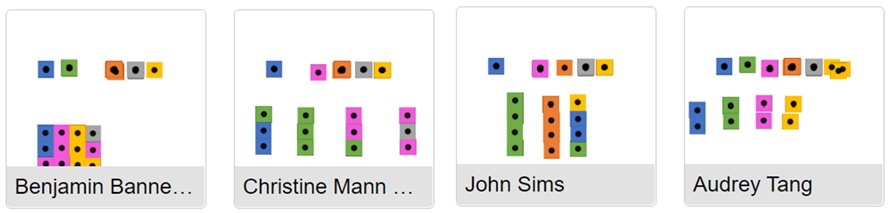While most students correctly leveled out the towers as shown in Benjamin and Christine’s work, some misconceptions arose. A few students made three even towers instead of four (John Sims’s work), which changed the number of groups from four to three. In this case, the student may not have understood that the height of towers may change but not the number of towers. Other students created four towers with the same number of cubes (Audrey Tang’s work). This means that either they did not begin with towers of different heights, and hence did not go through the process of “leveling out,” or their process of leveling out meant removing/adding blocks from the task rather than shifting them to another tower. Desmos Task: Balance of Weights We adapted Desmos’s Make It Balance task (Desmos, n.d.). First, students experiment by moving the triangle (balancing point) to balance two bears at fixed positions. The beam is shown with round support under both ends (i.e., Figure 2a). They click the “Check My Work” button to see the consequences of the triangle’s position; i.e., the supports on both sides are removed. If the balancing point is not centered, the beam sinks under the weight of the furthest bear (e.g., Figure 2b). The beam stays stable if the triangle is centered. In Figure 2, the left picture is the initial student screen, and the right picture shows a sample of student work. Notice that there can be only one position that results in a balanced beam for this activity–the midpoint between the two bears (i.e., Figure 2c). Figure 2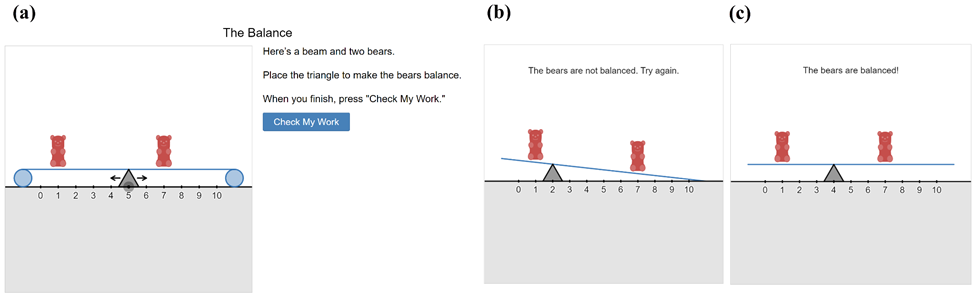Placing the Triangle to Balance Two Bears             Second, a beam and a fixed balance point are given, and students try to balance the beam by positioning the two bears. As before, they can use the “Check My Work” button to see the consequences of their work. We showed students various answers from their peers and facilitated a discussion about which one was correct. They concluded that in this activity there can be multiple ways to balance the bears. Figure 3 shows the student's initial screen (Gladys West, leftmost picture) and different ways of placing the bears in balance. It is interesting to note that no student placed the bears at 4 and 6. Figure 3 Placing two bears to Make Them Balance Third, students were given three bears on a beam and asked to explain a strategy they used to balance the bears (see Figure 4). They may draw to show their thinking but the activity is no longer interactive, which requires them to think more abstractly. Many students recognized that “I would have the triangle closer to the two bears to even out the weight as much as possible.” But they struggled to formally form a strategy to say exactly where the triangle needed to be moved in order for the bears to balance. One student came close by stating, “I balanced the bears by seeing what would be the equal distance between the single bear on the left to the two bears on the right.” Figure 4 Balancing Three Bears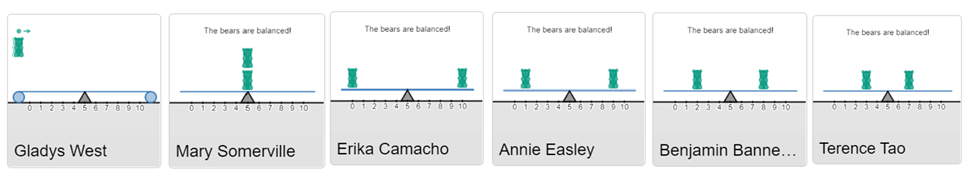Third, students were given three bears on a beam and asked to explain a strategy they used to balance the bears (see Figure 4). They may draw to show their thinking but the activity is no longer interactive, which requires them to think more abstractly. Many students recognized that “I would have the triangle closer to the two bears to even out the weight as much as possible.” But they struggled to formally form a strategy to say exactly where the triangle needed to be moved in order for the bears to balance. One student came close by stating, “I balanced the bears by seeing what would be the equal distance between the single bear on the left to the two bears on the right.” Figure 4 Balancing Three Bears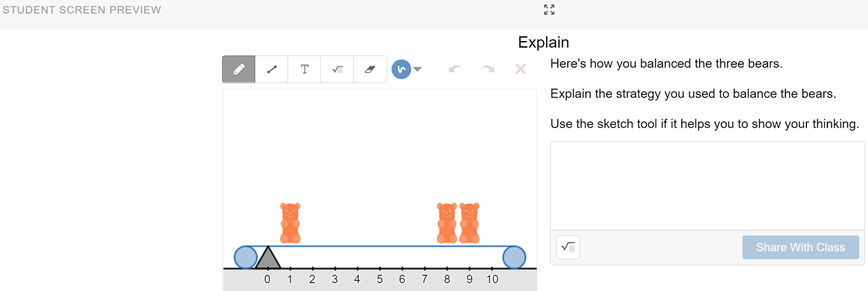After discussing this Balancing Three Bears task, we showed students the following sketch (Figure 5) and asked them to notice or wonder about how someone might use this sketch to support their explanation of the task. Many students then noticed that adding “adding the distance of the two bears together equals the distance to the one bear on the left.” We then moved from informal mathematics language to formal responses such as the triangle/fulcrum being the mean and  “distance from the triangle/fulcrum” being the deviation from the mean. Figure 5 Notice and Wonder of A Sketch Finally, to check students’ understanding of the mean as balancing weights, we gave students a sketch and asked them to decide, with reasoning, which set of bears are balanced. Figure 6 displays the student's initial screen on the left and a sample of student work on the right. While most students selected orange (the correct answer) and generally shared valid reasoning, fewer gave detailed responses. For example, many stated that the orange bears are balanced because “It is the same number of spaces on both sides.” A few students included more mathematical thinking in their responses, stating that the orange bears are balanced because “the added sum for the distance away from the fulcrum is same for both sides.” Figure 6 Determine Which Set of Bears is Balanced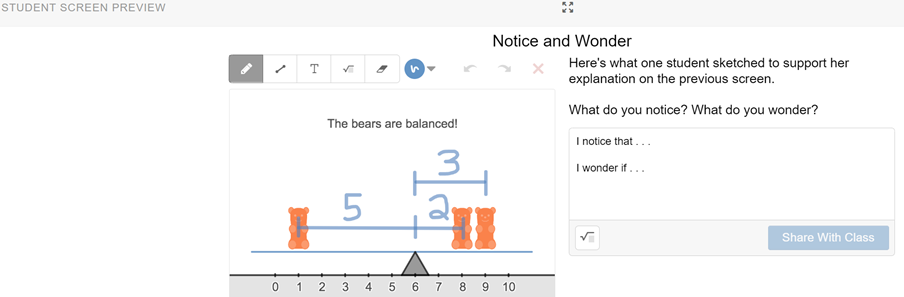Lessons Learned We plan to continue using these tasks in Desmos post-pandemic because we can notice our students’ thinking processes, work, and conceptions on the Desmos dashboard. The instant access to students’ work means we can discuss different strategies and conceptions with the whole class and revise tasks for future classes.             The misconceptions found in the Leveling Out task (John Sims’ and Audrey Tang’s work in Figure 1) might be due to misunderstanding the instructions of the task. To help with this, we may demonstrate how to build four towers of the required heights on Desmos first and emphasize leveling out the heights of these given towers, rather than creating new towers. For the Balance Weights task, students seemed to have more challenges balancing three bears. So we plan to give students more time to explore this activity and build formal mathematical language in their reasoning.    References American Statistical Association & National Council of Teachers of Mathematics [ASA & NCTM]. (2013). Preparing PreK-12 teachers of statistics: A joint position statement. Retrieved from  https://www.nctm.org/Standards-and-Positions/Position-Statements/Preparing-Pre-K-12-Teachers-of-Statistics Association of Mathematics Teacher Educators [AMTE]. (2016).  AMTE’s mission and goals. Retrieved from http://amte.net/about/mission Association of Mathematics Teacher Educators. (2017). Standards for Preparing Teachers of Mathematics, Raleigh, NC: Author. Beckmann, S. (2018). Mathematics for elementary school teachers (5th ed). Boston, MA: Pearson/Addison-Wesley. Conference Board of the Mathematical Sciences. (2012). The mathematical education of teachers II. Providence, RI and Washington, DC: Authors. Desmos. (2020). What we do. https://www.desmos.com/about Desmos. (n.d.). Make It Balance. Middle School Statistics. https://teacher.desmos.com/activitybuilder/custom/59de912a3f06a210c73513fa?collections=featured-collections%2C5da8a6474d5c010a4455b470 GAISE College Report ASA Revision Committee. (2016). Guidelines for Assessment and Instruction in Statistics Education College Report 2016. Retrieved from http://www.amstat.org/education/gaise Franklin, C., Kader, G., Mewborn, D., Moreno, J., Peck, R., Perry, M., & Scheaffer, R. (2007). Guidelines for assessment and instruction in statistics education (GAISE) report. Alexandria, VA: American Statistical Association. Maher, E.M., & Nguyen, H. (2021). Building Proof Bridges Interactively with Desmos. Reflections. Georgia Council of Teachers of Mathematics, 14(3). Seaton, J. (2020). Eli Luberoff – CEO and Founder of Desmos. The University of British Columbia, Vancouver Campus. https://virtual.educ.ubc.ca/wp/etec522/2020/02/01/eli-luberoff-ceo-and-founder-of-desmos/ Find a printable PDF version of this article here.